Name:    Scientific Notation Practice Quiz

Multiple Choice
Identify the choice that best completes the statement or answers the question.

1.

The radius of Earth is 6 370 000 m. Express this measurement in proper scientific notation.
 a.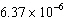m b.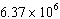m c.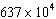m d.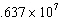m

2.

Which kind of graph would be best to use with these data?
M&M’s™ in a bag
 Color Orange Brown Yellow Green Blue Red Percent 20 13 14 16 24 13
 a. Line graph c. Bar graph b. Circle graph d. None of the above

3.

Solve (2 ´ 104) ´ (4 ´ 10–12) and express in scientific notation
 a. 8 ´ 108 cm b. 6 ´ 10–8 cm c. 8 ´ 10–8 cm d. 8 ´ 10–48 cm

4.

The diameter of a carbon atom is 0.000 000 000 154 m. What is this number expressed in scientific notation?
 a. 1.54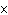10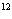m b. 1.5410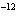m c. 1.5410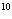m d. 1.5410m

5.

The expression of 0.6795 km in scientific notation is ____.
 a. 67.9510km b. 6.79510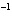km c. 6.79510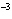km d. 6.79510km e. 679510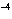km

6.

What is the result of multiplying 310by 410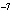?
 a. 1210b. 1210c. 1.210d. 1.2107.

The expression of 5008 km in scientific notation is ____.
 a. 5.00810km c. 5.00810km b. 50.0810km d. 5.00810km

8.

Solve:  (810) ¸ (410)?
 a. 210b. 210c. 210d. 210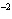True/False
Indicate whether the statement is true or false.

9.

A circle graph is most commonly used when the data is in the form of percentages.

10.

In most line graphs, the dependent variable is placed along the X-axis.

11.

A measurement of 5.685 x 10-6 is equivalent to 0.0000005685.

12.

A measurement of 2.79 x 104 is equivalent to 27,900.